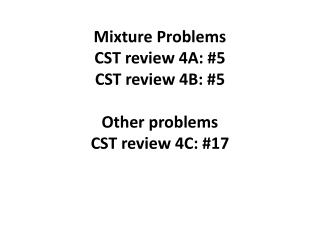DownloadDownload PresentationMixture Problems CST review 4A: #5 CST review 4B: #5 Other problems CST review 4C: #17

# Mixture Problems CST review 4A: #5 CST review 4B: #5 Other problems CST review 4C: #17

Télécharger la présentation## Mixture Problems CST review 4A: #5 CST review 4B: #5 Other problems CST review 4C: #17

- - - - - - - - - - - - - - - - - - - - - - - - - - - E N D - - - - - - - - - - - - - - - - - - - - - - - - - - -
##### Presentation Transcript

1. Mixture ProblemsCST review 4A: #5CST review 4B: #5Other problemsCST review 4C: #17

2. CST 4A, #5 The mechanic has 300 mL of battery acid solution that is 60% acid. He must add water to this solution to dilute it so that it is only 45% acid. How much water should be added? .60(300) + 0= .45(300 + x) 180 = 135 + .45x -135 = -135 45 = .45x x = 100 ml of water

3. CST 4B, #5 How many liters of a 70% alcohol solution must be added to 50 liters of a 40% alcohol solution to produce a 50% alcohol solution? Total sol = x + 50 .70x + .40(50) = .50(x + 50) .70x + 20= .50x + 25 .20x = 5 20x = 500 x = 25

4. CST 4C, #6 A health food store sells a mixture of peanuts and roasted almonds. Peanuts sell for \$3.50/lb and almonds sell for \$4.75/lb. How many pounds of each should be mixed to make 20 lbs. of this snack worth \$4.00/lb.? x + y = 20 3.50x + 4.75y = 4(x + y) 1000 = 125y 3.50x + 4.75y= 4x + 4y y = 8 x = 20 - 8 .5x = .75y 50x = 75y x = 12 x = 20 - y 50(20 - y) = 75y 12 lb peanuts 8 lb almonds 1000 - 50y = 75y

5. CST 4C, #17 x x x 160 cm2 14 14 – 2x 20 – 2x x 20 A picture frame measures 20 cm by 14 cm. The picture, inside the frame, takes up 160 square centimeters. Find the width of the frame. (20 – 2x)(14 – 2x) = 160 4(x - 15)(x - 2)= 0 x = 15, 2 280 – 68x + 4x2 = 160 x = 2 x can’t be 15 4x2 - 68x + 280= 160 14 – 2x = 14 – 2(2) = 10 4x2 - 68x + 120= 0 Width is 10, length is 20 – 2x = 20 – 2(2) =16 4(x2 - 17x + 30)= 0